Courses

# Solved Examples - Solutions, Class 12, Chemistry | EduRev Notes

## Class 12 : Solved Examples - Solutions, Class 12, Chemistry | EduRev Notes

The document Solved Examples - Solutions, Class 12, Chemistry | EduRev Notes is a part of the Class 12 Course Chemistry Class 12.
All you need of Class 12 at this link: Class 12

Example 1. The molarity of 20% (W/W) solution of sulphuric acid is 2.55 M. The density of the solution is:
(a) 1.25 g cm-3
(b) 0.125 g L-1
(c) 2.55 g cm-3
(d) unpredictable
Ans. (a)
Solution.
Volume of 100 g of solution = 100/d ml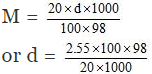= 1.249 ≈ 1.25

Example 2. The density of a solution containing 13% by mass of sulphuric acid is 1.09 g/mL. Calculate the molarity and normality of the solution-
(a) 1.445 M
(b) 14.45 M
(c) 144.5 M
(d) 0.1445 M
Ans. (a)
Solution.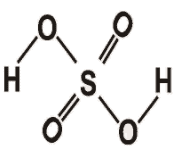H2SO4

Volume of 100 gram of the solution = 100/d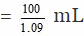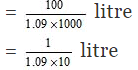Number of moles of H2SO4 in 100 gram of the solution = 13/98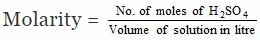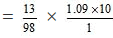= 1.445 M

Example 3. Calculate the molarity of pure water (d = 1g/L)
(a) 555 M
(b) 5.55 M
(c) 55.5 M
(d) None
Ans. (c)
Solution.
Consider 1000 mL of water
Mass of 1000 mL of water
= 1000 × 1 = 1000 gram
Number of moles of water = 100/18
= 55.5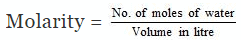= 55.5/1
= 55.5 M

Example 4. Calculate the quantity of sodium carbonate (anhydrous) required to prepare 250 ml of 0.1 M solution-
(a) 2.65 gram
(b) 4.95 gram
(c) 6.25 gram
(d) None
Ans. (a)
Solution.
We know that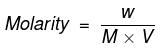where;
W = Mass of Na2CO3 in gram
M = Molecular mass of Na2CO3 in grams = 106
V = Volume of solution in litres = 250/1000 = 0.25
Molarity = 1/10
Hence, = 1/10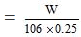or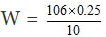= 2.65 gram

Example 5. The freezing point of a 0.08 molal aqueous solution of NaHSO4 is -0.372°C. The dissociation constant for the reaction
HSO4- ⇌ HSO42-is
(a) 4 × 10-2
(b) 8 × 10-2
(c) 2 × 10-2
(d) 1.86 × 10-2
Ans. (a)
Solution.
ΔTf = Kf × m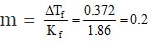This means that total molal conc. of all particles is 0.2.
NaHSO→ Na+ HSO4-
0.08                         0.08
HSO4  H+ + SO42-
(0.08 - x)       x           x
The net particle concentration
0.087 = (0.08 - x) + x + x = 0.2
or x = 0.04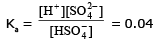Example 6. Find the molality of H2SO4 solution whose specific gravity is 1.98 g ml-1 and 95% by volume H2SO4
(a) 7.412
(b) 8.412
(c) 9.412
(d) 10.412
Ans. (c)
Solution.
H2SO4 is 95% by volume
wt. of H2SO4 = 95g
Vol of solution = 100ml
Moles of H2SO4 = , and weight of solution = 100 × 1.98 = 198 g
Weight of water = 198 - 95 = 103 g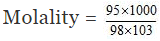= 9.412
Hence molality of H2SO4 solution is 9.412

Example 7. Calculate molality of 1 litre solution of 93% H2SO4 by volume. The density of solution is 1.84 gm ml-1
(a) 9.42
(b) 10.42
(c) 11.42
(d) 12.42
Ans. (b)
Solution.
Given H2SO4 is 93% by volume
wt. of H2SO4 = 93g
Volume of solution = 100ml
weight of solution = 100 × 1.84 gm
= 184 gm
wt. of water = 184 - 93 = 91 gm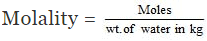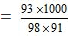= 10.42

Example 8. Suppose 5gm of CH3COOH is dissolved in one litre of Ethanol. Assume no reaction between them. Calculate molality of resulting solution if density of Ethanol is 0.789 gm/ml.
(a) 0.0856
(b) 0.0956
(c) 0.1056
(d) 0.1156
Ans. (c)
Solution.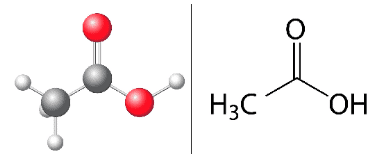Fig: Acetic acid structure

Wt. of CH3COOH dissolved = 5g
Eq. of CH3COOH dissolved = 5/60
Volume of ethanol = 1 litre = 1000ml.
Weight of ethanol = 1000 × 0.789 = 789g
Molality of solution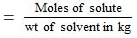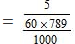= 0.1056

Example 9. Calculate the molarity and normality of a solution containing 0.5 gm of NaOH dissolved in 500 ml. solution-
(a) 0.0025 M, 0.025 N
(b) 0.025 M, 0.025 N
(c) 0.25 M, 0.25 N
(d) 0.025 M, 0.0025 N
Ans. (b)
Solution.
Wt. of NaOH dissolved = 0.5 gm
Vol. of NaOH solution = 500 ml
Calculation of molarity
0.5 g of NaOH = 0.5/40 moles of NaOH
[∴ Mol. wt of NaOH = 40]
= 0.0125 moles
Thus 500 ml of the solution contain NaOH = 0.0125 moles
1000 ml of the solution contain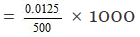= 0.025 M
Hence molarity of the solution = 0.025 M
Calculation of normality
Since NaOH is monoacidic;
Eq. wt. of NaOH = Mol. wt. of NaOH = 40
0.5 gm of NaOH = 0.5/40 gm equivalents = 0.0125 gm equivalents
Thus 500 ml of the solution contain NaOH = 0.0125 gm equ.
1000 ml of the solution contain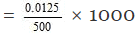Hence normality of the solution = 0.025 N

Example 10. Calculate the molality and mole fraction of the solute in aqueous solution containing 3.0 gm of urea per 250 gm of water (Mol. wt. of urea = 60).
(a) 0.2 m, 0.00357
(b) 0.4 m, 0.00357
(c) 0.5 m, 0.00357
(d) 0.7m, 0.00357
Ans. (a)
Solution.
Wt. of solute (urea) dissolved = 3.0 gm
Wt. of the solvent (water) = 250 gm
Mol. wt. of the solute = 60
3.0 gm of the solute = 3.0/60 moles = 0.05 moles
Thus 250 gm of the solvent contain = 0.05 moles of solute
1000 gm of the solvent contain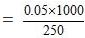= 0.2 moles
Hence molality of the solution = 0.2 m
In short,
Molality = No. of moles of solute/1000 g of solvent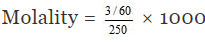= 0.2 m
Calculation of mole fraction
3.0 gm of solute = 3/60 moles = 0.05 moles
250 gm of water = 250/18 moles = 13.94 moles
Mole fraction of the solute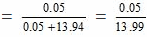= 0.00357

Example 11. A solution has 25% of water, 25% ethanol and 50% acetic acid by mass. Calculate the mole fraction of each component.
(a) 0.50, 0.3, 0.19
(b) 0.19, 0.3, 0.50
(c) 0.3, 0.19, 0.50
(d) 0.50, 0.19, 0.3
Ans. (d)
Solution.
Since 18 g of water = 1 mole
25 g of water = 25/18 = 1.38 mole
Similarly, 46 g of ethanol = 1 mole
25 g of ethanol = 25/46 = 0.55 moles
Again, 60 g of acetic acid = 1 mole
50 g of acetic acid = 50/60 = 0.83 mole
Mole fraction of water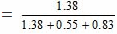= 0.50
Similarly, Mole fraction of ethanol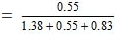= 0.19
Mole fraction of acetic acid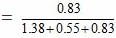= 0.3

Example 12. 15 gram of methyl alcohol is dissolved in 35 gram of water. What is the mass percentage of methyl alcohol in solution?
(a) 30%
(b) 50%
(c) 70%
(d) 75%
Ans. (a)
Solution.
Total mass of solution = (15 35) gram = 50 gram
mass percentage of methyl alcohol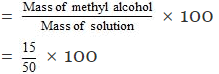= 30%

Example 13. Calculate the masses of cane sugar and water required to prepare 250 gram of 25% cane sugar solution?
(a) 187.5 gram, 62.5 gram
(b) 62.5 gram, 187.5 gram
(c) 162.5 gram, 87.5 gram
(d) None of these
Ans. (b)
Solution.
Mass percentage of sugar = 25
We know that
Mass percentage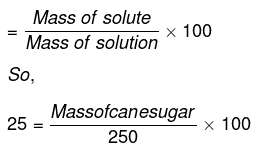or Mass of cane sugar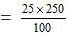= 62.5 gram
Mass of water = (250 - 62.5) = 187.5 gram

Offer running on EduRev: Apply code STAYHOME200 to get INR 200 off on our premium plan EduRev Infinity!

,

,

,

,

,

,

,

,

,

,

,

,

,

,

,

,

,

,

,

,

,

,

,

,

,

,

,

;